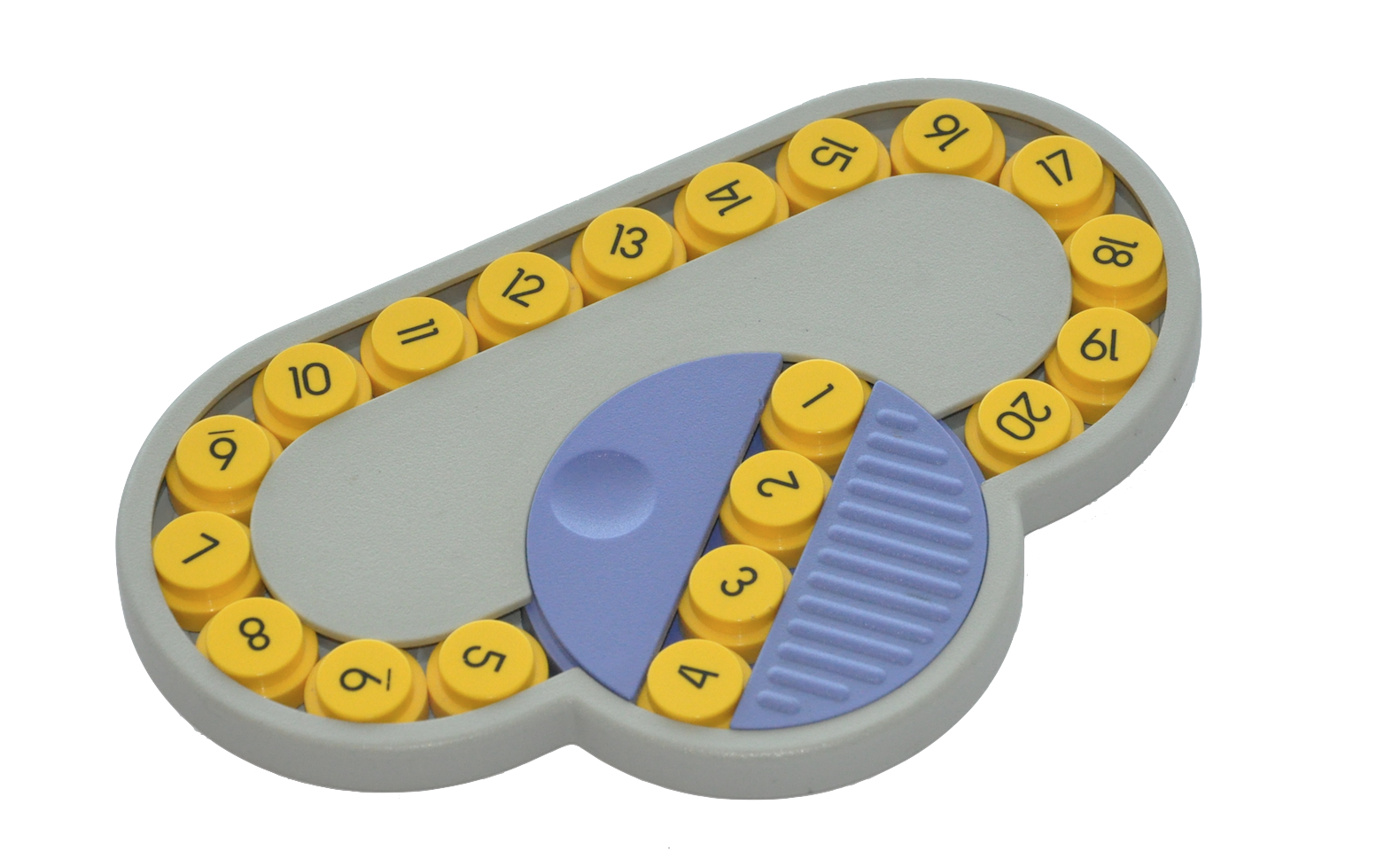## Oval Track puzzleThis puzzle consists of 20 numbered round pieces in one long looped track. You can slide all the pieces around the loop, or use the turntable in the loop (this is the purple circle which contains disks 1 through 4), which can rotate any four adjacent pieces so that they will be in reverse order. The goal is to mix up the ordering of the pieces, and then place the pieces back in numerical order.

Here is a very basic applet for the Oval Track puzzle: applet.
This was inspired by the applet written by Jaap Scherphuis © ( Jaap's Puzzle Page):

The basic legal moves of the Oval Track puzzle are $R$, and $T$, where $R$ denotes a clockwise rotation of numbers around the track, where each number moves one space, and $T$ denotes a rotation of the turntable.The permutations corresponding to the legal moves $R$, and $T$ are as follows: $$\begin{array}{l} R = (1,2,3,4,5,6,7,8,9,10,11,12,13,14,15,16,17,18,19,20) \\ T = (1,4)(2,3) \end{array}$$ Note that $T^{-1}=T$. This is due to the fact that spinning the turntable in either direction achieves the same result.

The Oval Track puzzle group $OT$ can be represented as a subgroup of the Symmetric group $S_{20}$ generated by these two permutations. $$OT = \langle R, T \rangle.$$

### Fundamental Puzzle Move Sequences

Without any knowledge of special move sequences it is possible to solve pieces $20$ through $5$, since there is enough wiggle room in the puzzle. Try this with the applet above.

Getting the last four pieces into place can be a bit more tricky. To solve the puzzle at this stage it is useful to have move sequences that result in moving only a few pieces at a time. We can produce a $2$-cycle (see below), but notice it is a fairly long move sequence (in Lecture 15 of the notes we show that this is the shortest possible). This means you would only want to use the $2$-cycle when it is the last thing to do to solve the puzzle, otherwise it would be best to use the $3$-cycle.

### $2$-cycle: $\sigma_2 = (TR^{-1})^{17} = (1,3)$### $3$-cycle: $\sigma_3 = [R^{-3},T]^2 = (1,7,4)$In the case of the $3$-cycle, to use this you'll need to identify the three disks you want to cycle, move these disks to positions $1$, $4$ and $7$ (and remember the sequence of moves you used to do this, call the seqnce $\beta$), then apply $\sigma_3$. Finally, undo the sequence of moves $\beta$ by applying $\beta^{-1}$.

### SageMath

We can define $OT$ in SageMath as follows.

### Variations of the Oval Track Puzzle

The turntable move can be changed to create a variety of oval track puzzles. Here are a few variations. The following variation adds orientation to the disks. The turnable move flips over the disks (rather then rotating them). White disks are "up", black disks are "down."